## Topic 10.4: Secant Method (Examples)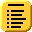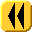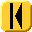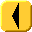Introduction Notes Theory HOWTO Examples Engineering Error Questions Matlab Maple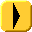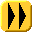# Example 1

As an example of the secant method, suppose we wish to find a root of the function f(x) = cos(x) + 2 sin(x) + x2. A closed form solution for x does not exist so we must use a numerical technique. We will use x0 = 0 and x1 = -0.1 as our initial approximations. We will let the two values εstep = 0.001 and εabs = 0.001 and we will halt after a maximum of N = 100 iterations.

We will use four decimal digit arithmetic to find a solution and the resulting iteration is shown in Table 1.

Table 1. The secant method applied to f(x) = cos(x) + 2 sin(x) + x2.

n xn − 1 xn xn + 1 |f(xn + 1)| |xn + 1 - xn|
10.0-0.1-0.51360.15220.4136
2-0.1-0.5136-0.61000.04570.0964
3-0.5136-0.6100-0.65140.00650.0414
4-0.6100-0.6514-0.65820.00130.0068
5-0.6514-0.6582-0.65980.00060.0016
6-0.6582-0.6598-0.65950.00020.0003

Thus, with the last step, both halting conditions are met, and therefore, after six iterations, our approximation to the root is -0.6595 .